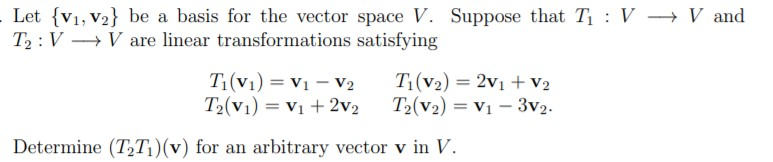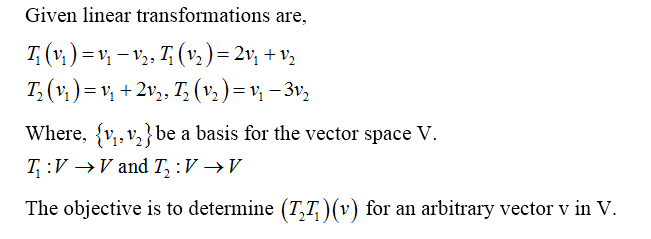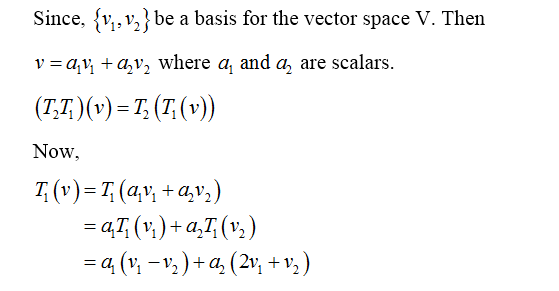# Let {v1, V2} be a basis for the vector space V. Suppose that T : V - V andT2 : V V are linear transformations satisfyingT1(V1) = V1 – V2T2(V1) = Vị + 2v2T(v2) = 2v1 + v2T2(v2) = V1 – 3v2.%3DDetermine (T,T1)(v) for an arbitrary vector v in V.

Question
31 views

6help_outlineImage TranscriptioncloseLet {v1, V2} be a basis for the vector space V. Suppose that T : V - V and T2 : V V are linear transformations satisfying T1(V1) = V1 – V2 T2(V1) = Vị + 2v2 T(v2) = 2v1 + v2 T2(v2) = V1 – 3v2. %3D Determine (T,T1)(v) for an arbitrary vector v in V. fullscreen
check_circle

star
star
star
star
star
1 Rating
Step 1Step 2...

### Want to see the full answer?

See Solution

#### Want to see this answer and more?

Solutions are written by subject experts who are available 24/7. Questions are typically answered within 1 hour.*

See Solution
*Response times may vary by subject and question.
Tagged in